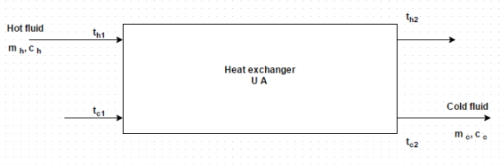# Test: Heat Flux Through A Cylindrical Wall And Plate

## 10 Questions MCQ Test Heat Transfer for Engg. | Test: Heat Flux Through A Cylindrical Wall And Plate

Description
This mock test of Test: Heat Flux Through A Cylindrical Wall And Plate for Chemical Engineering helps you for every Chemical Engineering entrance exam. This contains 10 Multiple Choice Questions for Chemical Engineering Test: Heat Flux Through A Cylindrical Wall And Plate (mcq) to study with solutions a complete question bank. The solved questions answers in this Test: Heat Flux Through A Cylindrical Wall And Plate quiz give you a good mix of easy questions and tough questions. Chemical Engineering students definitely take this Test: Heat Flux Through A Cylindrical Wall And Plate exercise for a better result in the exam. You can find other Test: Heat Flux Through A Cylindrical Wall And Plate extra questions, long questions & short questions for Chemical Engineering on EduRev as well by searching above.
QUESTION: 1

### When two fluids of the heat exchanger are separated by a plane wall, the thermal resistance comprises (i) Convection resistance due to the fluid film at the inside surface (ii) Conduction resistance (iii) Convection resistance due to the fluid film at the outside surface Identify the correct option

Solution:

It consists of all the above i.e. convection resistance and conduction resistance.

QUESTION: 2

### Figure represents the block diagram of a heat exchanger. There were some aspects in the design and performance analysis of a heat exchanger. Identify the correct oneSolution:

The structure of the heat exchanger transfers heat from the hot fluid to the coolant.

QUESTION: 3

### The heat loss from unpainted aluminum side of a house has been calculated on the presumption that overall coefficient of heat transfer is 5 W/m 2 K. Later, it was discovered that the air pollution levels are such that fouling factor on this side is of the order of 0.0005 m2 K/W. Find overall heat transfer coefficient of dirty side

Solution:

R = 1/U DIRTY – 1/U CLEAN.

QUESTION: 4

What is the value of fouling factor for engine exhaust?

Solution:

Its unit is m2 hr K/kcal and it represents the reciprocal of the scale coefficient i.e. heat transfer.

QUESTION: 5

What is the value of fouling factor for industrial liquids?

Solution:

Its unit is m2 hr K/kcal and it represents the reciprocal of the scale coefficient i.e. heat transfer.

QUESTION: 6

After being in service for a period of six months, a heat exchanger transforms 10% less heat than it does what new. Determine the effective fouling factor in terms of its clean overall heat transfer coefficient. It may be presumed that the heat exchanger operates between the same temperature differentials and that there is no change in the effective surface area due to scale build up

Solution:

CLEAN/Q DIRTY = U CLEAN/U DIRTY.

QUESTION: 7

In a counter flow heat exchanger, water flowing through a tube of 10 cm inner diameter is heated by steam condensing on the outside of the tube. The convective film coefficient on the water and steam side are estimated to be 12000 and 20000 k J/m2 hr degree. Neglecting tube thickness and its resistance to heat flow, workout the overall heat transfer coefficient for the heat exchanger

Solution:

U = 1/h + h o.

QUESTION: 8

A heat exchanger to preheat oil for a furnace was designed without considering the possibility of scale formation, and the overall heat transfer coefficient based on the fuel oil side was 3200 k J/m2 hr degree. What would be the corrected coefficient of heat transfer if a fouling factor of 0.00025 m2 hr degree/k J for the fuel oil is taken into account?

Solution:

U = 1/ (R + 1/h S).

QUESTION: 9

A copper pipe (k = 350 W/m K) having inner diameter of 1.75 cm and 2.0 cm outside diameter conveys water and the oil flows through the annular passage between this pipe and a steel pipe. On the water side, the film coefficient is 4600 W/m2 K and the fouling factor is 0.00034 m2 K/W. The corresponding values for the oil side are 1200 W/mK and 0.00086 m2K/W. Estimate the overall heat transfer coefficient between the oil and water

Solution:

A fouling factor represents the reciprocal of the scale coefficient i.e. heat transfer.

QUESTION: 10

Unit of fouling factor is

Solution:

The fouling factors are used in the design of heat exchangers. It is the overall heat transfer coefficient.